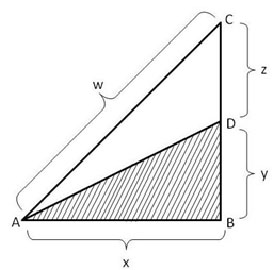# TANCET 2008 Quant Question 9 : Geometry

This geometry practice question appeared as a part of the quantitative reasoning or aptitude section of the TANCET 2008 MBA. Question 9 of a total of 20 questions that appeared in this section in TANCET 2008. This one is a medium level difficulty question and tests the following concepts: area of triangles and Pythagoras Theorem.

## Question

If the shaded area is one half the area of triangle ABC and angle ABC is right angle, then the length of line segment AD is1. $$frac{1}{2}$w 2. c 3. $\sqrt {{2x}^{2}+{z}^{2}}$ 4. $\sqrt {{w}^{2}-{3y}^{2}}$ 5. $\sqrt {{y}^{2}+{z}^{2}}$ Correct Answer Choice$4). $$sqrt {{w}^{2}-{3y}^{2}}$ ## Explanatory AnswerThe area of triangle ABD is half the area of triangle ABC. Both triangles have the same base AB. So, the altitude BD of triangle ABD will be half the altitude BC of triangle ABC. So, z = y. AD is the hypotenuse of the triangle ABD. So, AD = $\sqrt {{y}^{2} + {x}^{2}}$....$1)

In triangle ABC, AC2 = w2 = x2 + (y + z)2
But y = z, so we can rewrite the expression as w2 = x2 + (y + y)2
Or w2 = x2 + 4y2
Or x2 = w2 - 4y2

Substitute the value of x2 in (1): $$sqrt {{y}^{2}+{w}^{2}-{4y}^{2}}$ = $\sqrt {{w}^{2}-{3y}^{2}}$ The correct answer is Choice$4).

## Online TANCET MBA CourseTry it Free!

Register in 2 easy steps and start learning in 5 minutes!